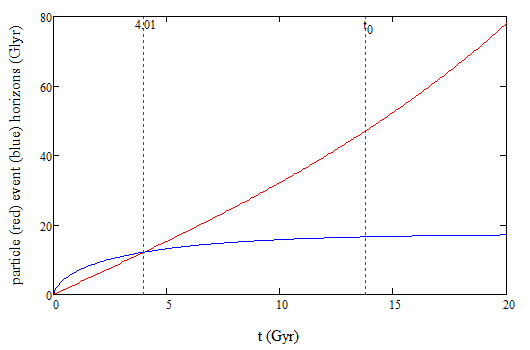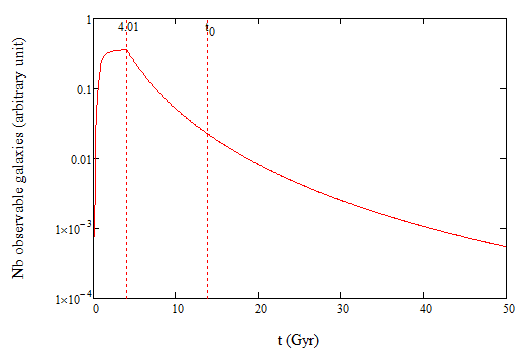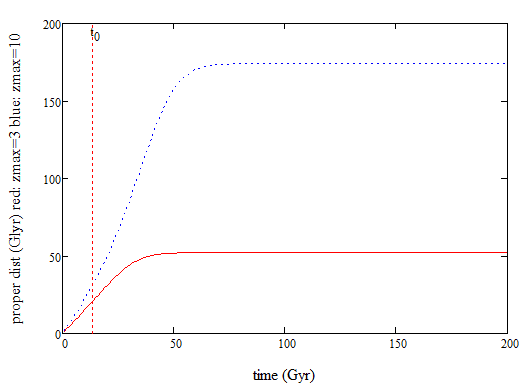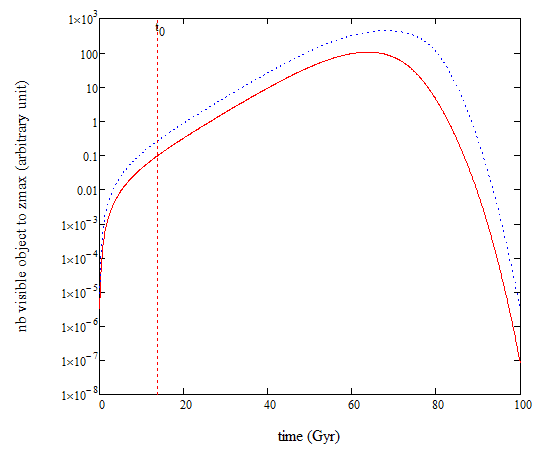# ##\Lambda##CDM: Evolution of the population of galaxies

• I
The number of visible galaxies is to vanish in the long-term future with the ##\Lambda##CDM model (book and papers of L. Krauss et al). I am interested in a quantitative study on how the population of galaxies decreases; I could not find one so I do it myself:

The scale factor: ##\displaystyle\frac{a(t)}{a_0}=(\frac{\Omega_{m0}}{\Omega_{\Lambda0}})^{^{^{1/3}}}\sinh^{^{2/3}}(\frac{t}{t_{\Lambda}})## with ##t_\Lambda=\displaystyle\frac{2}{\sqrt{3\Lambda c^2}}##, ##\Omega_x## the densities, and ##\Lambda## the cosmological constant.

The particle horizon: ##d_p(t)=a(t)\displaystyle\int_{0}^{t}\frac{cdt^\prime}{a(t^\prime)}##
The event horizon: ##d_E(t)=a(t)\displaystyle\int_{t}^{\infty}\frac{cdt^\prime}{a(t^\prime)}##
These horizons are shown in the figure:The two horizons cross at t=4.01 Gyr.

Let be ##h(t)## the smallest of the two horizons: ##h(t)=\min(d_p(t),d_E(t))##. The number of visible galaxies is then: ##V(t)\sim \Omega_m(a(t))h(t)^3## and this is shown on the figure below:The number of visible galaxies was maximum at 4.1 Gyr when the two horizons cross. Today it has decreased by a factor of 16, and at t=37.2 Gyr, it will have diminished by another factor of 16.
Is my calculation correct?

Bandersnatch
The number of visible galaxies is to vanish in the long-term future with the LCDM model (book and papers of L. Krauss et al).
In your earlier threads it was pointed out to you that this isn't true, unless you have a very specific meaning in mind (that you don't seem to be using here). Since you're back with essentially the same question, can you please indicate what did you find unconvincing in the arguments given so far? Or at least define what you mean by 'visible' galaxy, and explain why do you think the intersection of the two horizons has anything to do with it. If you think Krauss is making an argument to the contrary, please provide a specific reference, so that we can discuss it.

Bounded objects are not affected by the expansion, so the Local Supercluster will stay observable forever, as said in Krauss & Starkman 2000.

About Davis & Lineweaver 2004: I understand the Hubble sphere is not a horizon, but in a de Sitter universe, the Hubble sphere is the same as the event horizon. And in the far future, the LambdaCDM is to become a de Sitter universe. So I am not sure about their point about Krauss & Starkman in Appendix B13.

During inflation, a part of the universe that was causally connected (so was "observable") became stretched beyond the event horizon and is unobservable now. I gather that the same will happen with the LambdaCDM when it will become a de Sitter.

Bandersnatch
Can you also explain what you mean by a galaxy vanishing (or equivalently, being observable)? That is, when you want to count the number of visible galaxies at a given cosmic time, do you mean galaxies that are within the event horizon at that moment?; galaxies whose light reaches the observer at that moment?
I can't reconcile either of these with what you wrote above, so maybe you mean something else?

So I am interested in counting the galaxies that are observable at t, i.e., whose light reaches the observer at t.

I wonder what happens when the expansion makes the galaxy crosses the event horizon... when it crosses the particle horizon the redshift becomes infinite, but what happens when the event horizon is smaller than the particle horizon?

Bandersnatch
So I am interested in counting the galaxies that are observable at t, i.e., whose light reaches the observer at t.

I wonder what happens when the expansion makes the galaxy crosses the event horizon... when it crosses the particle horizon the redshift becomes infinite, but what happens when the event horizon is smaller than the particle horizon?
Ok. So if that's what you're after, then you should only be looking at the largest comoving extent (i.e. the base) of the past light cone, which is the same comoving distance as the particle horizon.

That these are the same distance (they're symmetrical on the conformal diagram) means that a galaxy crossing the particle horizon doesn't get redshifted to infinity - on a contrary, it only then becomes first observable (but not at the present state - at its earliest state). And it always grows, so the galaxy count always increases, albeit at ever slower rates.

Crossing the EH is never an event that can be observed - the light from the crossing event will take infinite time to get to the observer. You can calculate that a galaxy should have crossed the EH by some time t, but what you observe of that galaxy will always and forever be images from the past of that event. No observer can ever see the crossing of the horizon, so it can't remove galaxies from sight.

This is true whether we consider de Sitter, or a matter-dominated expansion approaching de Sitter.

The only sense in which removal happens is through gradual dimming of redshifted objects, until they can no longer be detected. But it isn't disappearing behind a horizon. It's a 'I no longer have sufficiently sensitive instruments' case, so considering horizon diagrams can't give you the answer you're looking for.

With the caveat that my understanding of inflation is cursory, I believe that the above is the case here too - it's not that the inflationary period ever disappeared anything from the initially causally connected region beyond the inflationary event horizon; it's that the observables were redshifted to oblivion, together with any matter and energy present.

What you could to do for this project instead, I think, is assume some maximum detection capability, and see when galaxies reach redshifts larger than that detection capability. This would introduce an arbitrary 'technological horizon' at some z value, that at some point in the future would stop the number of visible galaxies from increasing (since any later addition to the census would have higher z), before starting to reduce their number (as the already observable ones shift into higher z's).

I'm guessing Krauss goes for this meaning when he writes about only being able to see our local supercluster in the far future. With any non-infinite detection capabilities there will come a time in finite future, when every galaxy moving with the Hubble flow will end up beyond the detectability threshold.

But again, not much to do with the two horizons per se.

••mbond, Ibix and Keith_McClary
Bandersnatch
but what happens when the event horizon is smaller than the particle horizon?
I seem have skipped over this part - if you take another look back at our earlier discussion, with the graphs, you'll recall that this has no particular meaning, as the two horizons describe largely unrelated concepts.
It's important to remember that a galaxy crossing either of the two horizons doesn't directly affect the observer at the same cosmic time.
For the event horizon it's because information of that event will be received in the far (infinite) future, so it's not something the observer can care about now.
For the particle horizon it's because it marks when the light emitted by >observer< at t=0 reaches that galaxy (which is also when the light from that galaxy at t=0 reaches the observer), so this being outside the EH doesn't bother the observer (they see the light coming in, not moving out).

•mbond
PeterDonis
Mentor
2020 Award
when it crosses the particle horizon the redshift becomes infinite
That's what happens when it crosses the event horizon. Note that the crossings are in opposite directions: an object crosses the particle horizon inward (i.e., it transitions from not being visible to being visible--and this happens not "now", but just after the Big Bang--i.e., when an object has just crossed the particle horizon and become visible, we see it as it was just after the Big Bang); but an object crosses the event horizon outward (i.e., it transitions from being visible to not being visible).

The EH today is at 5.0 Gpc, z=1.8, so my calculation is completely wrong.

Here is a new one: I consider the proper distance to a given redshift as a function of t:
##d(t)=\displaystyle\int_{0}^{z_{max}}\frac{cdz}{H(z)}##
with ##\displaystyle 1+z(t)=\frac{a(t)}{a(t^\prime)}##, a generalization of ##\displaystyle 1+z=\frac{a_0}{a(t^\prime)}##.
One has ##H(t^\prime)=\displaystyle\frac{2}{3t_\Lambda}\frac{1}{\tanh(\frac{t^\prime}{t_\Lambda})}=\frac{2}{3t_\Lambda}\sqrt{\frac{(1+z)^3}{\sinh^2(t/t_\Lambda)}+1}## and the graph:The number of visible galaxies, ##V(t)\sim \Omega_m(a(t))d(t)^3##, is shown in the figure:So the number of visible galaxies up to zmax=3 or 10 is to increase by 1000 in the next 50 Gyr, and will sharply decrease after that.

The same calculation with a dust universe, ##a\sim t^{2/3}##, ##\Omega a^3## constant, yields ##V(t)\sim t##.

•Bandersnatch
Bandersnatch